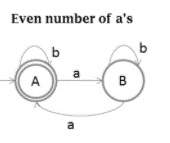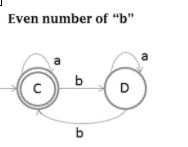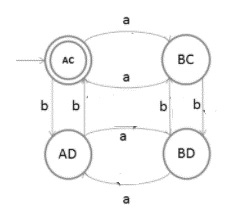# Explain the cross product method process in DFA

The cross product method process in the deterministic finite automata (DFA) is explained below −

Let a's DFA diagram has m number of states and b's DFA diagram has n number of states the cross product m x n will have mxn states.

Languages represented by even number of ‘a’ and even number of ‘b’ are given below −

L1 = {ε, baa, aa, aba, aab, aaaa, ... }

L2 = {ε bb, abb, bab, bba, ...}

After cross product we will find the DFA as mentioned below −

As, L = {ab, aab, abb, aaab, ...}

Example

Let’s taken two DFAs

• Even number of a's
• Even number of b's

The DFA for even number of a’s is as follows −The DFA for even number of b’s is as follows −Cross product of two languages is as follows −

{A, B} X {C, D} = {AC, AD, BC, BD}

The state transition diagram for the cross product of two languages is given below −Example

Determine the transition for each state (AC, AD, BC, BD) and for each input (a,b).

## Explanation

Follow the steps given below to find out the transition for each state and for each input.

• Step 1

State AC and input a

a's DFA diagram − A → a → B

b's DFA diagram − C → a → C

Result − AC→ a → BC

• Step 2

State AC and input b

a's DFA diagram − A → b → A

b's DFA diagram − C → b → D

Result − AC→ b → AD

• Step 3

State BC and input a

a's DFA diagram − B → a → A

b's DFA diagram − C → a → C

Result − BC→ a → AC

• Step 4

State BC and input b

a's DFA diagram − B → b → B

b's DFA diagram − C → b → D

Result − BC→ b → BD

• Step 5

State BD and input a

a's DFA diagram − B → a → A

b's DFA diagram − D → a → D

Result − BD→ a → AD

• Step 6

State BD and input b

a's DFA diagram − B → b → B

b's DFA diagram − D → b → C

Result − BD→ b → BC

• Step 7

a's DFA diagram − A → a → B

b's DFA diagram − D → a → D

Result − AD→ a → BD

• Step 8